# SQL Operators

## Introduction

In this chapter, you will learn SQL operators in detail starting with SQL Operator types, their usages, and examples in SQL.

## SQL Operators

A SQL operator is a sign or symbol that can perform an operation between operands or between two or more than two expressions.

SQL operators can be broken down into the following categories:
1. Arithmetic Operators
2. Assignment Operators
3. Bitwise Operators
4. Comparison Operators
5. Logical Operators
6. Scope Resolution Operator
7. String Concatenation Operators
8. Unary Operators
9. Set Operators
Let us discuss step-by-step each of the operators listed above.

## SQL Arithmetic Operators

To perform a mathematical operation on one or more expressions of a numeric data type, like addition, subtraction, multiplication, division, and modulo division then we use an arithmetic operator.

The list of arithmetic operators are given below,

 Operator Meaning Example + Addition 15 + 2 = 17 - Subtraction 15 - 2 = 13 * Multiplication 15 * 2 = 30 / Division 15 / 2 = 7 % Modulo 15 % 2 = 1 (Reminder)

Example 1.1
1. declare @var1 int,
2. @var2 int,
4. @sub int,
5. @mul int,
6. @div int,
7. @rem int-- declaration of var1, var2, add,sub,mul,div
8.
9. set @var1=12;--assigning var1=12
10. set @var2=5;--assigning var1=12
11.
13. set @sub = @var1 - @var2;--Subtraction
14. set @mul = @var1 * @var2;--Multiplication
15. set @div = @var1 / @var2;--Division
16.
17. select @var1 as Variable1,
18. @var2 as Variable2,
20. @sub as Subtraction,
21. @mul as Multipication,
22. @div as division; --getting the result by using select
By using the code above you will get the following output,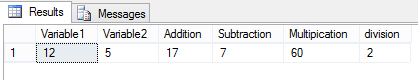Example 1.2

Suppose I have a table named TempTable in which I have 2 columns like a and b, and I have inserted some values of a and b as in the following,
1. --Creating Table
2. CREATE TABLE #TempTable(
3.    a int,
4.    b int
5. )
6. --Inseerting Values
7. INSERT INTO #TempTable VALUES(1,2),(12,8),(8,10),(17,3),(11,4),(15,8)
By using the query above you will get a new #TempTable such as the following,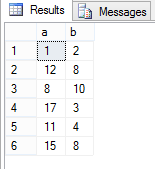Now you can also use arithmetic operators with your query like,
1. SELECT
2. as value1,
3. as value2,
4. (a + b) as ADDITION,
5. (a - b) as SUBTRACTION,
6. (a * b) as MULTIPLICATION,
7. (a / b) as DIVISION,
8. (a % b) as REMINDER
9. FROM #TempTable
The output of the above Query is,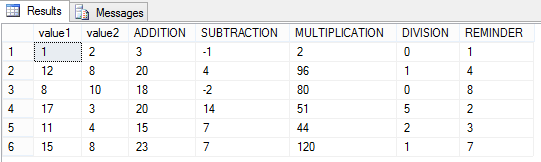## SQL Assignment Operator

The SQL assignment operator is used to assign the value to a variable. We usually see an assignment operator '='.

Example 2.1

In the following example, I am simply taking a variable and assigning a value.
1. --Declaring variable
2. declare @h int;
3.
4. --Assigning Value
5. set @h=15;
6.
7. --Getting the output with column name Assigned
8. select @h as Assigned
So simply run the code above in T-SQL and you will get the output: 15 in AssiginedColumn.

The same as in other languages like C, C++, Java, C#, and so on. in T-SQL we have Shorthand Assignment Operator. The shorthand assignment operators in SQL are +=, -=, *=, /=, %=. shorthand assignment operators are illustrated in the following table.

 Statement With Simple Assignment Operator Statement With Shorthand Assignment Operator a = a + b a+=b a = a – b a-=b a = a * b a*=b a = a / b a/=b a = a % b a%=b

The following are some examples of shorthand assignment operators.

Example 2.2
1. declare @i int;
2. set @i=15;
3. set @i+=10;
The output of the code above is 25 with column name Addition.

Example 2.3
1. declare @j int;
2. set @j=15;
3. set @j-=10;
4. select @j as Subtraction
The output of the code above is 5 with column name Subtraction.

Example 2.4
1. declare @k int;
2. set @k=15;
3. set @k*=10;
4. select @k as Multiplication
The output of the code above is 150 with column name Multiplication.

Example 2.5
1. declare @L int;
2. set @L=15;
3. set @L/=10;
4. select @L as Divide
The output of the code above is 1 with column name Divide.

Example 2.6
1. declare @m int;
2. set @m=15;
3. set @m%=10;
4. select @m as Reminder
The output of the code above is 5 with column name Reminder.

Example 2.7

Suppose you have a table of Employee_Info in which you have the 3 fields id, Name, Salary and inside it some data is available like,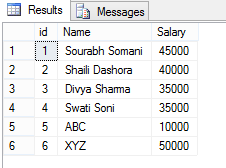Now if you fire a query like,
1. select id, Name, Salary=10000 from Employee_Info --assigning every value as 10000 Salary
Then every value of the salary will be set to 10000 and the output of the code above will be,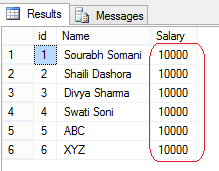## SQL Bitwise Operators

As in other languages (like C, C++, Java, C#, and so on), SQL also supports special operators that are the bitwise operators. Bitwise operators manipulate the data at the bit level. The operations are used to test and/or the bits. Bitwise operators are only applied for integer values they cannot be applied for float or doubled value.

There are four Bitwise operators available in T-SQL that are as follows,

 Operator Meaning & (Bitwise AND) Performs bitwise AND operation | (Bitwise OR) Performs bitwise OR operation ^ (Bitwise XOR) Performs bitwise Exclusive-OR operation ~ (Bitwise NOT) Performs bitwise Not operation

### Bitwise AND (&)

The Bitwise logical AND operation is performed between 2 integer values.

Example 3.1

Suppose there are the two integer numbers 2 and 3 and we want to perform 2 & 3 so first we convert 2 and 3 into the binary format. Then after the AND operation is performed on the bits then the bits will again be converted into the decimal format and that will be our output. The complete illustration is given below in the following figure,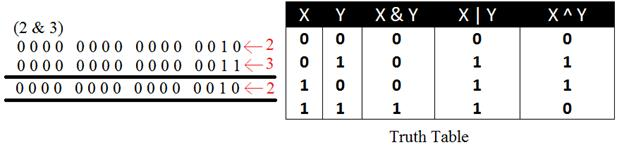### Bitwise OR ( | )

Bitwise logically OR operation is performed between 2 integer values.

Example 3.2

Suppose there are the two integer numbers 2 and 3 and we want to perform 2 | 3 so we first convert 2 and 3 into binary format. Then after the OR operation is performed on the bits then the bits will again be converted into the decimal format and that will be our output. The complete illustration is given below in the figure,### Bitwise XOR ( ^ )

The Bitwise logical Exclusive-OR operation is performed between 2 integer values.

Example 3.3

Suppose there are the two integer numbers 2 and 3 and we want to perform 2 ^ 3 so we first convert 2 and 3 into the binary format. Then after the XOR operation is performed on the bits then the bits will again be converted into the decimal format and that will be our output. The complete illustration is given below in the figure,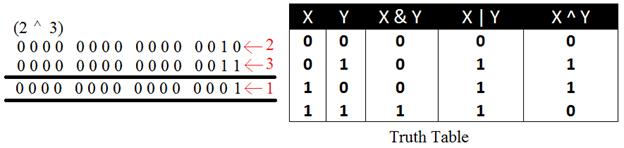### Bitwise Not ( ~ )

The Bitwise logical NOT operation is performed on only one integer value.

Example 3.4

Suppose we have the number 2 and we want to perform the operation ~2 then first 2 will be converted into binary format then after the not operation is performed on the bits then the bits will again be converted it into decimal format.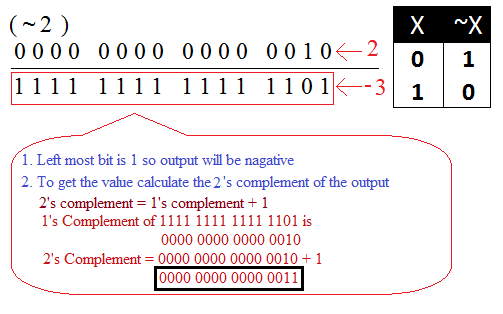Example 3.5

In the following example, I am writing a SQL query for examples 3.1, 3.2, 3.3, and 3.4 explained above.
1. declare @a int;
2. declare @b int;
3.
4. set @a=2;
5. set @b=3;
6.
7. select @a & @b as And_Operation,
8. @a | @b as Or_Operation,
9. @a ^ @b as Exclusive_Operation,
10. ~@a as Bitwise_NOT_A,
11. ~@b as Bitwise_NOT_B ;
The output of the preceding query will be,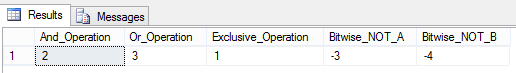Example 3.6

In this example, I am showing how to perform bitwise operations on a table. Suppose you have a temporary table in which you have the two columns val1 and val2, in which some data is present like,
1. Create table #MyTempTable(
2.    val1 int,val2 int
3. )
4. insert into #MyTempTable values(2,3),(5,3),(8,5),(9,4)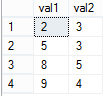You can perform bitwise operations with your query like,
1. select val1 as Value1,
2. val2 as Value2,
3. val1 & val2 as Bitwise_And_Operation,
4. val1 | val2 as Bitwise_OR_Operation,
5. val1 ^ val2 as Bitwise_XOR_Operation,
6. ~val1 as Bitwise_Not_Value1,
7. ~val2 as Bitwise_Not_Value2
8. from #MyTempTable
The output of the code above will be,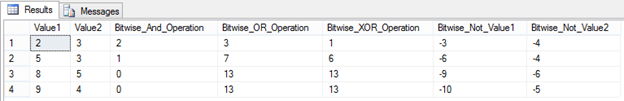## SQL Comparison Operators

The comparison operator is also called a Relational Operator. We often compare two quantities and depending on their relations, make a certain decision. For example, we may compare the age of two people, or the price of two items, or the salary of two people, and so on.

These types of comparisons are done by relational or comparison Operators. The list of Comparison operators available in T-SQL is given in the following table,

 Operator Meaning = Equal to < Less than > Greater than <= Less than equal to >= Greater than equal to <> Not equal to != Not equal to(Not ISO Standard) !< Not less than(Not ISO Standard) !> Not greater than(Not ISO Standard)

Example 4.1

Suppose you have a table #TempTable (temporary table) in which you have two columns val1 and val2. Some values are also inserted into it that are like,
1. --Creating a temporary table
2. create table #TempTable(
3. val1 int,
4. val2 int
5. )
6. --Inserting Data
7. insert into #TempTable values(8,10),(17,3),(11,4),(15,8),(8,9),(8,17),(8,8)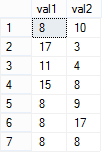Now I am showing you how can you fire a query for all comparison operators.

### For Equals (=)

1. select val1 as Value1,
2. val2 as Value2
3. from #TempTable
4. where val1=val2
Output

Where val1 and val2 will be the same that rows will be selected.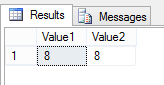### Less than (<)

1. select val1 as Value1,
2. val2 as Value2
3. from #TempTable
4. where val1<val2
Output

Where val1 will be less than the from val2, those rows will be selected.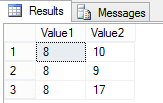### Greater than (>)

1. select val1 as Value1,
2. val2 as Value2
3. from #TempTable
4. where val1>val2
Output

Where val1 will be greater than from val2, those rows will be selected.### Less than or equals to (<=)

1. select val1 as Value1,
2. val2 as Value2
3. from #TempTable
4. where val1<=val2
Output

Where val1 will be less than or equal to from val2, those rows will be selected.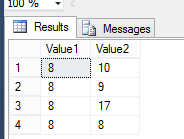### Greater than equals to (>=)

1. select val1 as Value1,
2. val2 as Value2
3. from #TempTable
4. where val1>=val2
Output

Where val1 will be greater than or equal to from val2, those rows will be selected.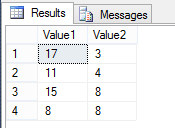### Not equals to (!=)

1. select val1 as Value1,
2. val2 as Value2
3. from #TempTable
4. where val1!=val2
Output

Where val1 will not be equal to from val2, those rows will be selected.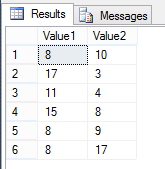### Not equals to (<>)

1. select val1 as Value1,
2. val2 as Value2
3. from #TempTable
4. where val1<>val2
Output

Where val1 will not be equal to from val2, those rows will be selected. The same output will be as for the Not equals to (!=)

### Not less than (!<)

1. select val1 as Value1,
2. val2 as Value2
3. from #TempTable
4. where val1!<val2
Output

Where val1 will not be less than from val2, those rows will be selected.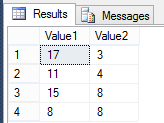### Not greater than (!>)

1. select val1 as Value1,
2. val2 as Value2
3. from #TempTable
4. where val1!>val2
Output

Where val1 will not be greater than from val2, those rows will be selected.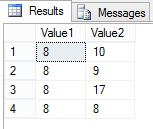## SQL Logical Operators

Logical operators in SQL are used to check some condition and returns a boolean value that may be true or false.

The list of logical operators of SQL is listed below in the table.

 Operator Description All Check for all the set of comparisons. AND Check that two values are both true. ANY Check for anyone's set of comparisons. BETWEEN Check between the range. EXISTS Check whether or not a sub-query has a row. IN Check a list of expressions. LIKE Match the patterns. NOT Reverse the value of a Boolean value. OR Check between two values and anyone should be true. SOME Check that some set of comparisons are true or not

Now let's see a -by-step explanation of all the logical operators:

### All

This operator returns true if and only if when all the set of comparisons are true. It compares the scalar value with a single column set of values.

Example 5.1

Suppose you have the two tables #tb1, #tb2 and you have inserted a Name and age in both tables like,
1. create table #tb1(
2.  Name varchar(20),
3.  age int
4.  )
5.  create table #tb2(
6.  Name varchar(20),
7.  age int
8.  )
Both tables will look such as in the following:

#tb1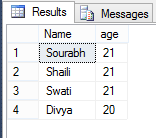#tb2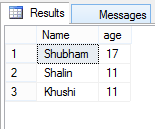Now to understand the ALL operator use the following query.
1. SELECT * FROM #tb1
2. WHERE age > All (SELECT age FROM #tb2)
So the output of the preceding query will be,Because all the sets of comparisons are true with respect to #tb2. But take a scenario where one age is greater in #tb2 with respect to all the ages of #tb1. Suppose I insert some data like this,
1. insert into #tb2 values('Monika Dashora',25)
After inserting the row #tb2 will become,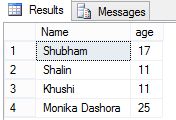Now again you fire the same query like,
1. SELECT * FROM #tb1
2. WHERE age > All (SELECT age FROM #tb2)
Then by using the query above you will get #tb1 as null. Because in the #tb2 we have the age of "monika dashora" as "25" and if it will be checked against #tb1 then the age will always be less then 25.

### ANY

Any operator returns true if one of the set comparisons are true. This operator also compares a scalar value with a single column set of values.

Example 5.2

Suppose you have the same table that we had in Example 5.1 which is #tb1 and #tb2 and you fire the following query,
1. SELECT * FROM #tb1
2. WHERE age > ANY (SELECT age FROM #tb2)
Then you will get the output as:Because the ANY operator compares a set of values not for all.

Note

The difference between ALL and ANY is,

ALL works like an AND so if all the comparisons are true then true is returned else it returns false, ANY works like an OR so if anyone comparison is true then it returns true.

### SOME

SOME and ANY are equivalent, both compare a single column's set of values.

### AND

This operator is applied between two expressions and can also be applied between more than two expressions. If all the expression's conditions are true then it returns true otherwise it returns false.

Example 5.3

Suppose we have the same table that we had in Example 5.1. Now to see how to use the AND operator see the following query,
1. select * from #tb2 where age>11 AND age<25
By using the query above you will get those tuples from the table (#tb2) that will be less than 25 but greater than 11 that will be,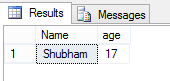### OR

This operator also can be applied between two and more than two expressions. If any condition is true then it will return true.

Example 5.4

Suppose we have the same table that we had in Example 5.1. Now to see how to use the OR operator see the following query:
1. --The following query return that tuples where age=11 or age=25
2. select * from #tb2 where age=11 OR age=25
By using the above query we will get the following output,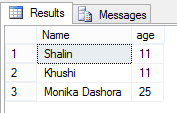### NOT

This operator reverses the value of a Boolean value.

Example 5.5

Suppose we have the same table that we had in Example 5.1. As I just said, the NOT operator reverses the output so I am using the AND operators example above, Example 5.3, but with the NOT operator.
1. -- Following code will give that tuple where age will be greater than 25 but not be less than 25
2. select * from #tb2 where age>11 AND NOT age<25
The output of the code above is,### Between

This operator returns the value true if and only if the operand is within the range. This operator works with the AND operator.

Example 5.6

Take the same table #tb2 of Example 5.1. To learn how to use the Between Operator execute the following query.
1. -- following query will return those tuples where age is between 11-17
2. select * from #tb2 where age Between 11 AND 17
The output of the preceding query is:You can also execute with the NOT operator so the output will be the reverse.
1. select * from #tb2 where age NOT Between 11 AND 17### Exists

The Exists operator will return true when the subquery contains any row.

Example 5.7

Suppose we have the same table that we had in Example 5.1. Now execute the following query,
1. --following query returns all the ages from the #tb1 if subquery will contain row
2. SELECT AGE FROM #tb1 WHERE EXISTS (SELECT AGE FROM #tb2);
By using the code above the output will be,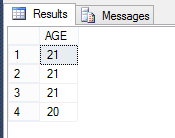Suppose you write the query as in the following,
1. SELECT AGE FROM #tb1 WHERE EXISTS (SELECT AGE FROM #tb2 where age>25);
By using the query above we will get the output as a null table because the sub-query doesn't have a row.

### IN

This operator returns true if the operand is equal to one of a list of expressions.

Example 5.8

Suppose we have the same table that we had in Example 5.1, and for the IN operator we can write the query like this,
1. -- This will return that tuples which have the age 21 and 18
2. SELECT * FROM #tb1 WHERE AGE IN ( 21, 18 );
The output will be,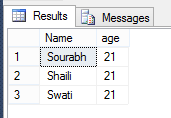### LIKE

This operator is used with the WHERE clause to search for the specified pattern in the column.

Example 5.9

Suppose we have a table #TempTable (temporary table) in which you have 2 columns named First_Name and Last_Name with some values inserted inside it that is as follows,
1. --String Operator
2. create table #TempTable(
3.    First_Name varchar(20),
4.    Last_Name varchar(20)
5. )
6. --Inserting Values inside the #TempTable
7. insert into #TempTable values
8. ('Sourabh''Somani'),
9. ('Shaili''Dashora'),
10. ('Swati''Soni'),
11. ('Divya''Sharma')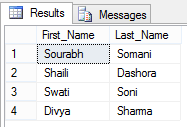You can use the LIKE query as in the following,
1. --1st query (It will select that tuple where First_Name start with 's' )
2. select * from #TempTable where First_Name like 's%'
3.
4. --2nd query (It will select that tuple where Last_Name start with 'd' )
5. select * from #TempTable where Last_Name like 'd%'
6.
7. --3rd query (It will select that tuple where in Last_Name contains 'o' )
8. select * from #TempTable where Last_Name like '%o%'
9.
10. --4th query (It will select that tuple where in Last_Name contains 'om' )
11. select * from #TempTable where Last_Name like '%om%'
Now from the code above, I will get output like this,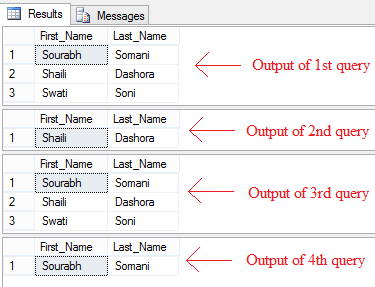## SQL Scope Resolution Operator

By using scope resolution operator (::) we can access static members of a compound datatype.

Example 6.1
1. DECLARE @hid hierarchyid;
2. SET @hid = hierarchyid::GetRoot();
3. SELECT @hid.ToString() as _Root;
Output

The code above shows how the scope resolution operator accesses the static member GetRoot() of type hierarchyid.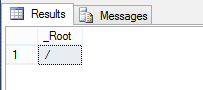## SQL String Concatenation Operators

The string concatenation operators concat two or more binary strings. There are the following string concatenation operators available:

 Operator Description + Concatenate the string += Concatenate the string % Wildcard character(s) matches [] Wildcard character(s) matches [^] Wildcard character(s), not matches _ Match with only one character

### + String Concatenation Operators

This operator concatenates two or more strings, characters, or columns into the single string.

Example 7.1

Suppose we have a table #TempTable (temporary table) in which you have 2 columns named First_Name and Last_Name and have some values inserted inside it that is as follows,
1. --String Operator
2. create table #TempTable(
3.    First_Name varchar(20),
4.    Last_Name varchar(20)
5. )
6. --Inserting Values inside the #TempTable
7. insert into #TempTable values
8. ('Sourabh''Somani'),
9. ('Shaili''Dashora'),
10. ('Swati''Soni'),
11. ('Divya''Sharma')Now I am writing the following query in which I am getting a full name from the first name and last name using the + string concatenation operator.
1. select
2. First_Name,
3. Last_Name,
4. (First_Name+' '+Last_Name) as Full_Name
5. from #Temptable
Output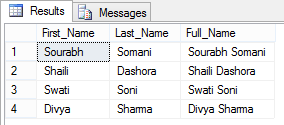### += String Concatenation Operators

This operator concatenates the strings to the result of the operation.

Example 7.2

Suppose I have the same table I had in the previous Example 7.1. Now I use the following query to concatenate the string with +=.
1. declare @Str varchar(20);
2. set @Str='Bittu ';
3. set @Str += (select Last_Name from #Temptable where First_Name='Sourabh');
4. insert into #Temptable(First_Name) values(@Str);
5.
6. select * from #Temptable -- after concatenate using (+=)
The output of the preceding query will be,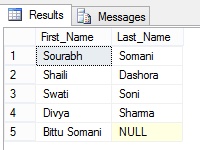### % Wildcard Character(s) match operator

This operator matches zero or more characters. This operator is mainly used in searching. It is used either as a prefix or suffix or for both sides as in the following:

%Charecter(s) or Charecter(s)% or %Charecter(s)%

Example 7.3

Suppose I have the same table that I had in Example 7.1 and I am searching as in the following,
1. --1st query (It will select that tuple where First_Name start with 's' )
2. select * from #TempTable where First_Name like 's%'
3.
4. --2nd query (It will select that tuple where Last_Name start with 'd' )
5. select * from #TempTable where Last_Name like 'd%'
6.
7. --3rd query (It will select that tuple where in Last_Name contains 'o' )
8. select * from #TempTable where Last_Name like '%o%'
9.
10. --4th query (It will select that tuple where in Last_Name contains 'om' )
11. select * from #TempTable where Last_Name like '%om%'
Now from the code above, I will get output like this,### [ ] Wildcard Character(s) match operator

This operator only matches a single character within the range specified inside the brackets. This operator is mainly used to match the pattern like a regular expression.

Example 7.4

To understand this operator let's create a table #TempTable (temporary table) with the 3 columns First_Name, LastName and Mobile_Number and then insert data into it like,
1. --Creating Temoporary table(#TempTable) with 3 columns
2. --First_Name
3. --Last_Name
4. --Mobile_Number
5. create table #TempTable(
6.    First_Name varchar(20),
7.    Last_Name varchar(20),
8.    Mobile_Number varchar(10)
9. )
10. --Inserting data into it
11. insert into #TempTable values
12. ('Sourabh''Somani',9314543761),
13. ('Shaili''Dashora',8892724222),
14. ('Swati''Soni',9928486447),
15. ('Divya''Sharma',7737334455)
So the #TempTable will look like,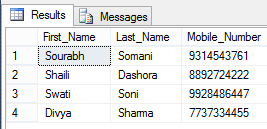After inserting the data to understand the [ ] wildcard character(s) match operator, let's execute a query like this,
1. -- select number which
2. --1st latter should be 8 or 9
3. --2nd latter should be between 3 to 8
4. select * from #TempTable where Mobile_Number like '[8-9][3-8]%'
After executing the query above the result of the query will be,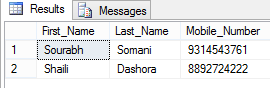### [^ ] Wildcard Character(s) not match operator

This operator is the opposite of the [ ] operator, this operator matches the single character that is not in the range that is specified in the brackets.

Example 7.5

Let's use the same table that we had in Example 7.4 and execute the following query.
1. --following query tells that select First_Name from the table where First_Name's first latter should not be 'd'
2. select * from #TempTable where First_Name like '[^d]%'
The output of the preceding query after execution will be,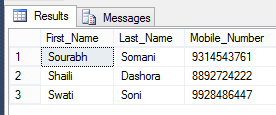### _ Wildcard Match one character operator

This operator only matches a single character in a string; this is also used to compare the strings.

Example

Let's use the same table that we had in Example 7.4 and execute the following query.
1. --Following query select that tuple that matches from
2. --s_a_l_
3. --or
4. --s_u_a_h
5. --here _ operator represents any character so in output tuple it should be verifry
6. --s<Any Character>a<Any Character>l<Any Character>
7. --or
8. --s<Any Character>u<Any Character>a<Any Character>h
9. select * from #TempTable where First_Name like 's_a_l_%' or First_Name like 's_u_a_h'
The output of the preceding query will be,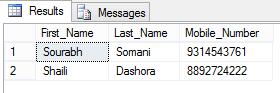## SQL Unary Operators

The unary operator is the operator that only takes a single operand in an expression or a statement. There are 3 unary operators available in SQL as listed in the following table:

 Operator Description + (Unary Plus) Positive numeric value - (Unary Minus) Negative numeric value ~ (Bitwise NOT) Provide the one's complement of the number

Example 8.1

The following is the unary minus and unary plus example.
1. declare @a int
2. declare @b int
3. set @a=-17
4. set @b=+8
5. select ABS(@a) as Absolute_Value_Of_a , @a as Actual_Value_Of_a,
6. ABS(@b) as Absolute_Value_Of_b , @b as Actual_Value_Of_b
Note

## SQL Set Operators

These operators combine the results from two or more queries into a single result set. There are three types of set operators available as listed below,

 Operator Description EXCEPT Returns any distinct value from the left query which does not belong in the right query. INTERSECT Returns distinct values from which are common in both the queries. UNION Combine the result of two and more queries into a single result set that belongs to all queries in the union.

### EXCEPT

This operator returns any distinct value form the left query that does not belong in the right query.

Example 9.1

Suppose there are the two tables Employee1 and Employee2 as in the following,
1. --1st Table
2. create table Employee1(
3.    EmpId int,
4.    First_Name varchar(20),
5. )
6. --2nd Table
7. create table Employee2(
8.    EmpId int,
9.    First_Name varchar(20),
10. )
The following values are inserted into it,
1. --Values for table 1
2. insert into Employee1 values
3. (1,'Sourabh'),
4. (2,'Shaili'),
5. (3,'Swati'),
6. (4,'Divya')
7.
8. --Values for table2
9. insert into Employee2 values
10. (1,'Sourabh'),
11. (2,'Shaili'),
12. (3,'Bittu')
If you execute an EXCEPT query like,
1. --EXCEPT  Query
2. (select * from Employee1)  EXCEPT  (select * from Employee2)
Output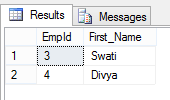### INTERSECT

This operator returns any distinct value that is common in both queries.

Example 9.2

In the above example if we use INTERSECT instead of EXCEPT then the output will be,
1. --Intersect Query
2. (select * from Employee1)  INTERSECT (select * from Employee2)
Output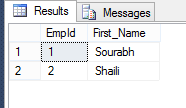Note

INTERSECT Operator is the inverse of EXCEPT.

### UNION

Combine the two queries into a single result set and no repetition will exist for UNION.

Example 9.3

In the above example if we use UNION instead of EXCEPT/INTERSECT then the output will be,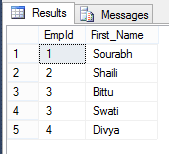### Rules for SET Operators

• The number of columns must be equal in both queries.
• The order of the columns must be the same in all queries.
• The Data Type must be compatible.

## Summary

In this chapter on SQL operators, we discussed various operators available in SQL. We also saw how to use these operators with SQL queries and the results of the queries.